# Non-Stationary Stochastic Multi-Armed Bandits¶

A well-known and well-studied variant of the stochastic Multi-Armed Bandits is the so-called Non-Stationary Stochastic Multi-Armed Bandits. I give here a short introduction, with references below. If you are in a hurry, please read the first two pages of this recent article instead (arXiv:1802.08380).

• The first studied variant considers piece-wise stationary problems, also referred to as abruptly changing, where the distributions of the $K$ arms are stationary on some intervals $[T_i,\ldots,T_{i+1}]$ with some abrupt change points $(T_i)$.

• It is always assumed that the location of the change points are unknown to the user, otherwise the problem is not harder: just play your favorite algorithm, and restart it at each change point.

• The change points can be fixed or randomly generated, but it is assumed that they are generated with a random source being oblivious of the user’s actions, so we can always consider that they were already generated before the game starts.

• For instance, Arms.geometricChangePoints() generates some change point if we assume that at every time step $t=1,\ldots,T]$, there is a (small) probability p to have a change point.

• The number of change points is usually denoted $L$ or $\Upsilon_T$, and should not be a constant w.r.t. $T$ (otherwise when $T\to\infty$ only the last section counts and give a stationary problem so it is not harder). Some algorithms require to know the value of $\Upsilon_T$, or at least an upper-bound, and some algorithms try to be efficient without knowing it (this is what we want!).

• The goal is to have an efficient algorithm, but of course if $\Upsilon_T = \mathcal{O}(T)$ the problem is too hard to hope to be efficient and any algorithm will suffer a linear regret (i.e., be as efficient as a naive random strategy).

• Another variant is the slowly varying problem, where the rewards $r(t) = r_{A(t),t}$ is sampled at each time from a parametric distribution, and the parameter(s) change at each time (usually parametrized by its mean). If we focus on 1D exponential families, or any family of distributions parametrized by their mean $\mu$, we denote this by having $r(t) \sim D(\mu_{A(t)}(t))$ where $\mu_k(t)$ can be varying with the time. The slowly varying hypothesis is that every time step can be a break point, and that the speed of change $|\mu_k(t+1) - \mu_k(t)|$ is bounded.

• Other variants include harder settings.

• For instance, we can consider that an adversarial is deciding the change points, by being adaptative to the user’s actions. I consider it harder, as always with adversarial problems, and not very useful to model real-world problems.

• Another harder setting is a “pseudo-Markovian rested” point-of-view: the mean (or parameters) of an arm’s distribution can change only when it is sampled, either from time to time or at each time step. It makes sense for some applications, for instance Julien’s work (in SequeL Inria team), but for others it doesn’t really make sense (e.g., cognitive radio applications).

TODO fix notations more precisely, include definitions! TODO what are the lower-bounds given in the more recent articles?

## Applications¶

TL;DR: the world is non stationary, so it makes sense to study this!

TODO write more justifications about applications, mainly for IoT networks (like when I studied multi-player bandits).

## Example of simulation configuration¶

A simple python file, configuration_nonstationary.py, is used to import the arm classes, the policy classes and define the problems and the experiments. The main.py file is used to import the configuration and launch the simulations.

For example, we can compare the standard UCB and Thompson algorithms, non aware of the non-stationarity, against the non-stationarity aware DiscountedUCB SWUCB, and the efficient DiscountedThompson algorithm.

We also included our algorithms Bernoulli-GLR-UCB using kl-UCB, and compare it with CUSUM-UCB and M-UCB, the two other state-of-the-art actively adaptive algorithms.

horizon = 5000
change_points = [0, 1000, 2000, 3000, 4000]
nb_random_events = len(change_points) - 1 # t=0 is not a change-point
list_of_means = [
[0.4, 0.5, 0.9], # from 0 to 1000
[0.5, 0.4, 0.7], # from 1000 to 2000
[0.6, 0.3, 0.5], # from 2000 to 3000
[0.7, 0.2, 0.3], # from 3000 to 4000
[0.8, 0.1, 0.1], # from 4000 to 5000
]

configuration = {
"horizon": horizon,    # Finite horizon of the simulation
"repetitions": 1000,  # number of repetitions
"n_jobs": -1,        # Maximum number of cores for parallelization: use ALL your CPU
"verbosity": 5,      # Verbosity for the joblib calls
# Environment configuration, you can set up more than one.
"environment": [     # Bernoulli arms with non-stationarity
{   # A non stationary problem: every step of the same repetition use a different mean vector!
"arm_type": Bernoulli,
"params": {
"listOfMeans": list_of_means,
"changePoints": change_points,
}
},
]
],
# Policies that should be simulated, and their parameters.
"policies": [
{ "archtype": klUCB, "params": {} },
{ "archtype": Thompson, "params": {} },
{ "archtype": OracleSequentiallyRestartPolicy, "params": {
"policy": klUCB,
"changePoints": change_points,
"list_of_means": list_of_means,
"reset_for_all_change": True,
"reset_for_suboptimal_change": False,
}}
{ "archtype": SWklUCB, "params": { "tau":  # formula from [GarivierMoulines2011]
2 * np.sqrt(horizon * np.log(horizon) / (1 + nb_random_events))
} },
{ "archtype": DiscountedklUCB, "params": { "gamma": 0.95 } },
{ "archtype": DiscountedThompson, "params": { "gamma": 0.95 } },
{ "archtype": Monitored_IndexPolicy, "params": {
"horizon": horizon, "policy": klUCB, "w": 150,
} },
{ "archtype": CUSUM_IndexPolicy, "params": {
"horizon": horizon, "policy": klUCB, "w": 150, "max_nb_random_events": nb_random_events, "lazy_detect_change_only_x_steps": 10, # Delta n to speed up
} } ] + [
{ "archtype": BernoulliGLR_IndexPolicy_WithDeterministicExploration,
"params": {
"horizon": horizon, "policy": klUCB_forGLR, "max_nb_random_events": nb_random_events,
"lazy_detect_change_only_x_steps": 10, # Delta n to speed up
"lazy_try_value_s_only_x_steps": 10, # Delta s
"per_arm_restart": per_arm_restart,
} }
for per_arm_restart in [True, False]
]
}


## How to run the experiments ?¶

You should use the provided Makefile file to do this simply:

# if not already installed, otherwise update with 'git pull'
git clone https://github.com/SMPyBandits/SMPyBandits/
cd SMPyBandits
make install         # install the requirements ONLY ONCE


Then modify the configuration_nonstationary.py file, to specify the algorithms you want to compare (use the snippet above for inspiration). And run with:

make nonstationary   # run and log the main.py script


There is a couple of different piece-wise stationary problems, that we implemented for our article, and you can use environment variables to modify the experiment to run. For instance, to run problems 1 and 2, with horizon T=5000, N=1000 repetitions, using 4 cores, run:

PROBLEMS=1,2 T=5000 N=1000 N_JOBS=4 DEBUG=False SAVEALL=True make nonstationary


## Some illustrations¶

Here are some plots illustrating the performances of the different policies implemented in this project, against various non-stationary problems (with Bernoulli only).

### History of means for this simple problem¶

We consider a simple piece-wise stationary problem, with $K=3$ arms, a time horizon $T=5000$ and $N=1000$ repetitions. Arm changes concern only one arm at a time, and there is $\Upsilon=4$ changes at times $1000,2000,3000,4000$ ($C_T=\Upsilon_T=4)$.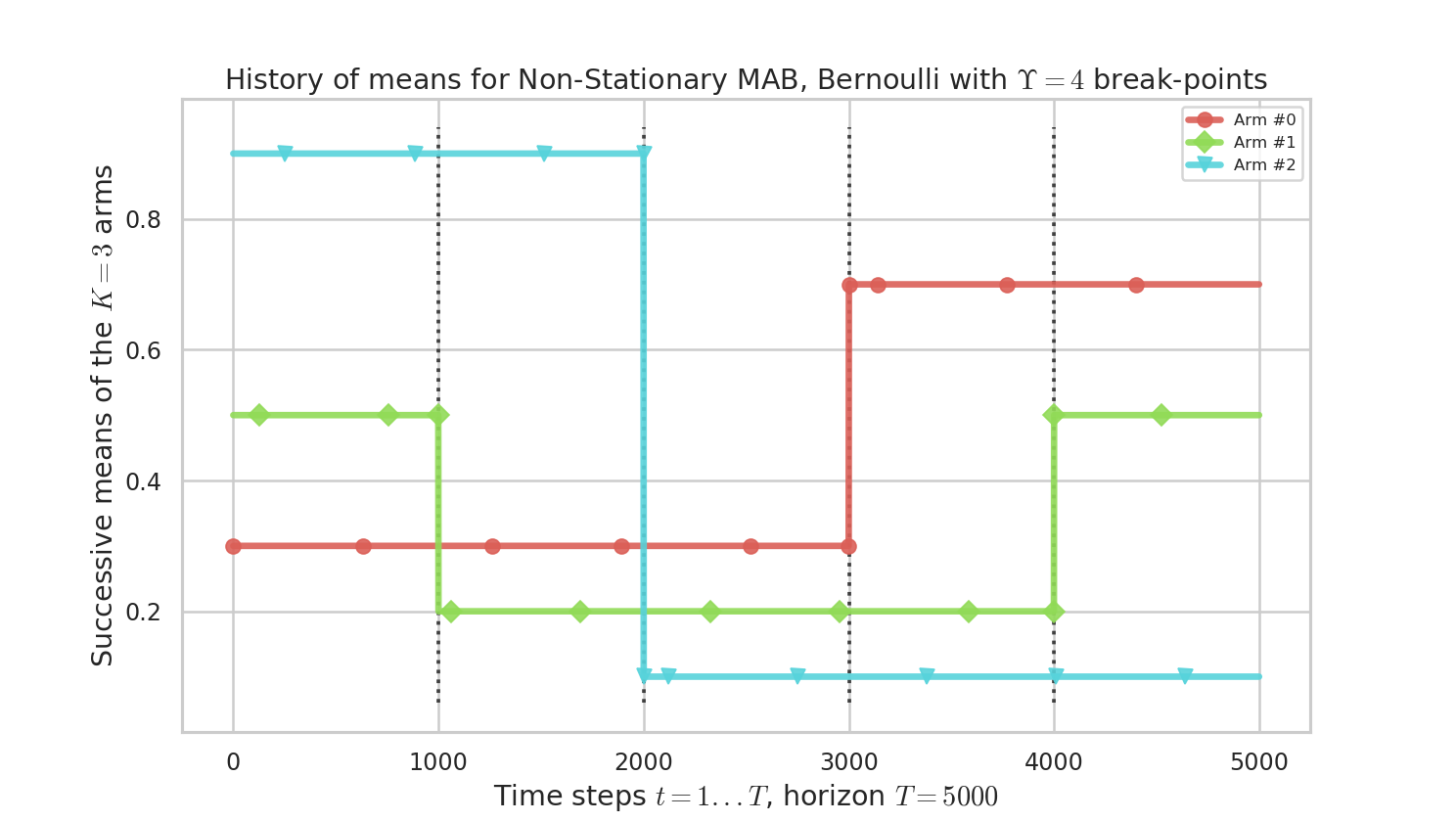Figure 1 : history of means $\mu_i(t)$ for the $K=3$ arms. There is only one change of the optimal arm.

The next figures were obtained with the following command (at the date of writing, 31st of January 2019):

PROBLEMS=1 T=5000 N=1000 N_JOBS=4 DEBUG=False SAVEALL=True make nonstationary


### Comparison of different algorithms¶

By using the configuration snippet shown above, we compare 9 algorithms. The plots below show how to perform. Our proposal is the GLR-klUCB, with two options for Local or Global restarts (Generalized Likelihood Ratio test + klUCB), and it outperforms all the previous state-of-the-art approaches.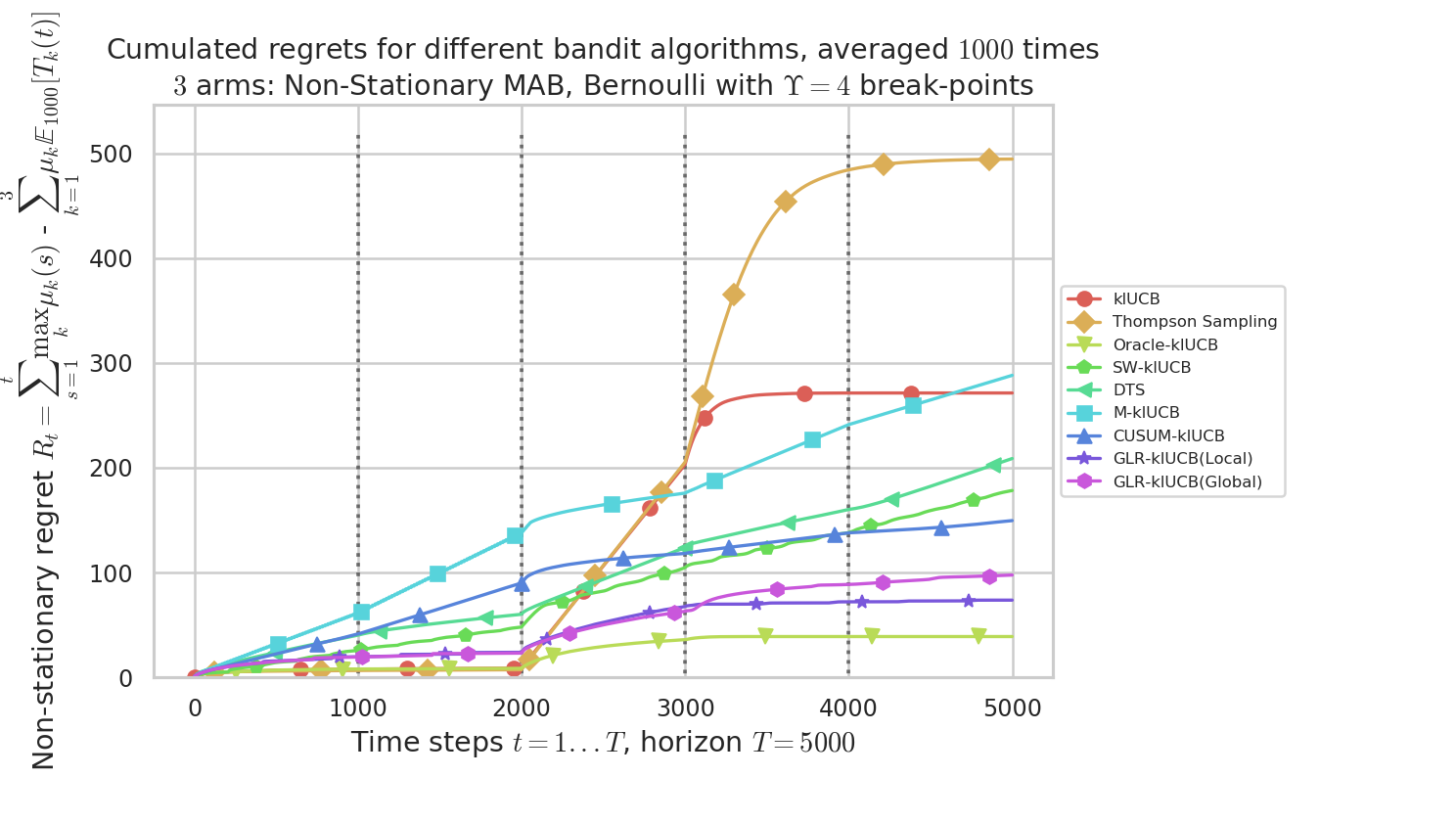Figure 2 : plot of the mean regret $R_t$ as a function of the current time step $t$, for the different algorithms.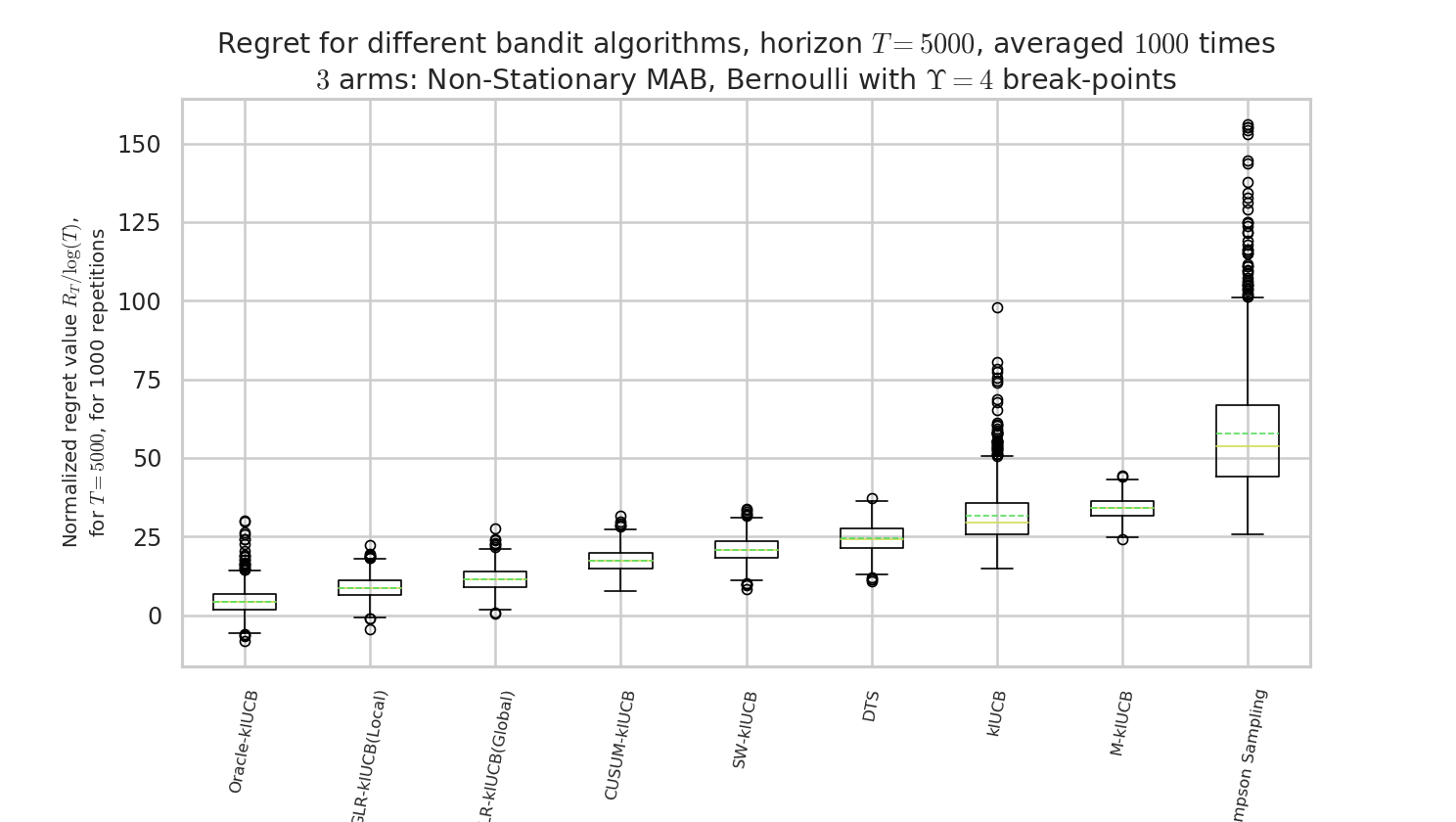Figure 3 : box plot of the regret at $T=5000$, for the different algorithms.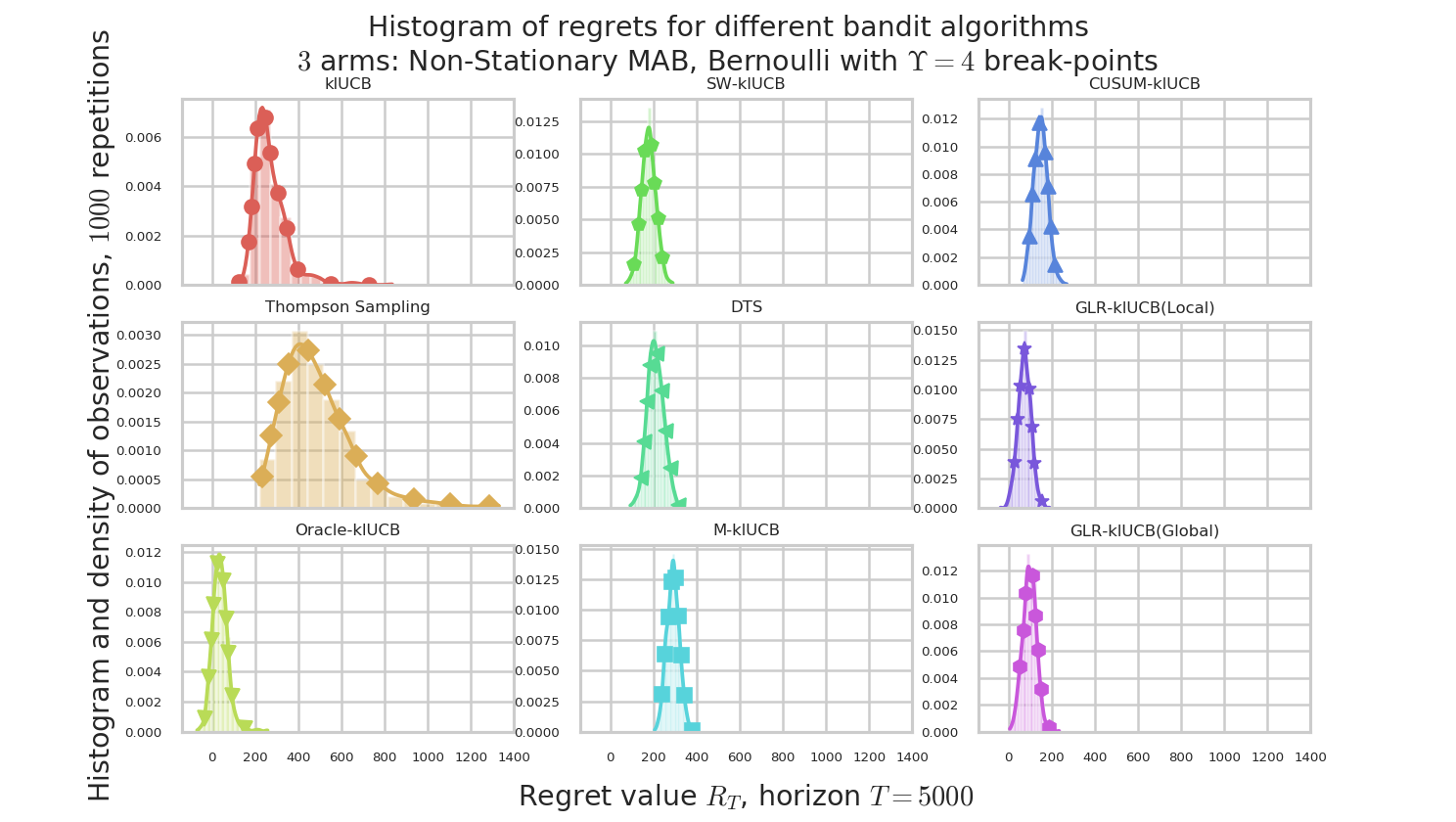Figure 4 : plot of the histograms of the regret at $T=5000$, for the different algorithms.

### Comparison of time and memory consumptions¶Figure 5 : comparison of the running times. Our approach, like other actively adaptive approach, is slower, but drastically more efficient!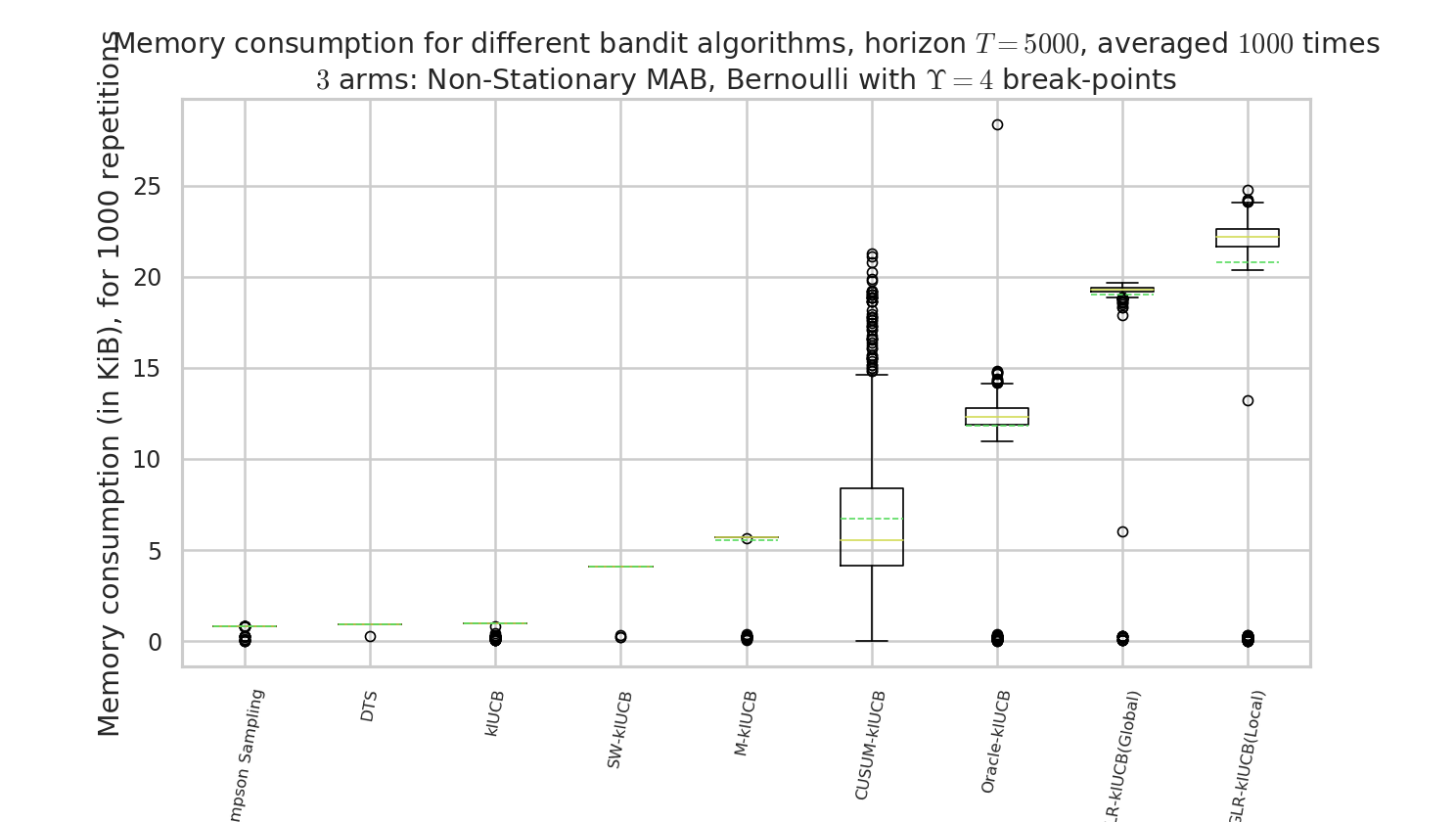Figure 6 : comparison of the memory consumption. Our approach, like other actively adaptive approach, is more costly, but drastically more efficient!

## Article?¶

Not yet! We are working on this! TODO